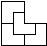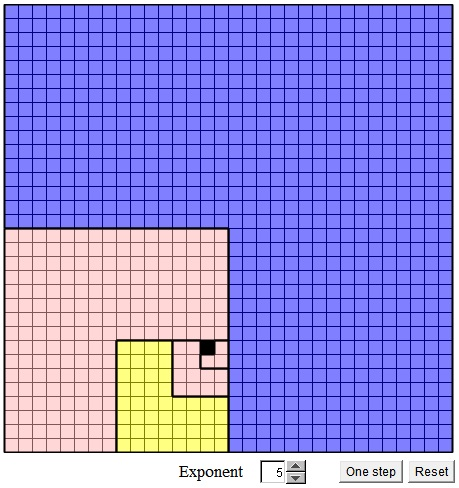Tromino as a Rep-tile

The applet below illustrates a proof of S. Golomb's theorem based on the fact that the L-shaped (or right tromino) is a rep-4 tile. (The term rep-tile has also been introduced by Golomb to describe a shape that is tiled by smaller copies of itself.)Theorem

A unit square has been removed from a 2n×2n board. The rest of the board can be tiled with L-shaped trominos.

By clicking anywhere in the applet you can define (and redefine) the missing square. The "exponent" parameter defines n, for a 2n×2n board. The number can be changed with a spin control at the bottom of the applet.

If you are reading this, your browser is not set to run Java applets. Try IE11 or Safari and declare the site https://www.cut-the-knot.org as trusted in the Java setup.What if applet does not run?

ProofA unit square has been removed from a 2n×2n board. The rest of the board can be tiled with L-shaped trominos.

Proof

Divide the board into 4 equal squares. The missing square belongs to one of the four. The other three form an L-shaped tromino, which, being a rep-4 tile, can be tiled by smaller copies of itself, which, in turn, can be tiled (if necessary) by smaller copies of themselves, and so on. Every size board can be treated in this manner. In particular, we can apply this step to the remaining square where the small missing square is located. The process will stop when n = 1, i.e., when a 2×2 square is reached that conisists of a single L-tromino and the missing square.

References

1. S. Golomb, Two Right Tromino Theorems, in The Changing Shape of Geometry, edited by C. Pritchard, Cambridge University Press, 2003
2. S. Golomb, Polyominoes, Princeton University Press, 1994• Covering A Chessboard With Domino
• Dominoes on a Chessboard
• Tiling a Chessboard with Dominoes
• Vertical and Horizontal Dominoes on a Chessboard
• Straight Tromino on a Chessboard
• Golomb's inductive proof of a tromino theorem
• Tromino Puzzle: Interactive Illustration of Golomb's Theorem
• Tiling Rectangles with L-Trominoes
• Squares and Straight Tetrominoes
• Covering a Chessboard with a Hole with L-Trominoes
• Tromino Puzzle: Deficient Squares
• Tiling a Square with Tetrominoes Fault-Free
• Tiling a Square with T-, L-, and a Square Tetrominoes
• Tiling a Rectangle with L-tetrominoes
• Tiling a 12x12 Square with Straight Trominoes
• Bicubal Domino
•